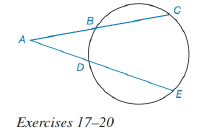Chapter 6.3, Problem 17E### Elementary Geometry for College St...

6th Edition
Daniel C. Alexander + 1 other
ISBN: 9781285195698

#### Solutions

Chapter
Section### Elementary Geometry for College St...

6th Edition
Daniel C. Alexander + 1 other
ISBN: 9781285195698
Textbook Problem
43 views

# For Exercises 17 to 20. See Theorem 6.3.6.Given: A B = 6 , B C = 8 , A E = 15 Find: DE.To determine

To find:

The value DE.

Explanation

Given that, AB=6,BC=8,AE=15.

The diagrammatic representation is given below,

Theorem:

If secant segments are drawn to a circle from an external point, then the products of the length of each secant with the length of its external segment are equal.

By using the theorem to get the following,

Also know that

AB+BC=AC6+8=ACAC=14

Substitute the values AB=6,AC=14,AE=15 in the above equation to get the following,

### Still sussing out bartleby?

Check out a sample textbook solution.

See a sample solution

#### The Solution to Your Study Problems

Bartleby provides explanations to thousands of textbook problems written by our experts, many with advanced degrees!

Get Started

#### Given that the point P(2, 4) lies on the line 2x + ky = 8, find k.

Applied Calculus for the Managerial, Life, and Social Sciences: A Brief Approach

#### Solve the equations in Exercises 126. x69x4=0

Finite Mathematics and Applied Calculus (MindTap Course List)

#### What is the value of (X + 1) for the following scores: 0, 1, 4, 2? a. 8 b. 9 c. 11 d. 16

Essentials of Statistics for The Behavioral Sciences (MindTap Course List)

#### The curvature of at t = 0 is:

Study Guide for Stewart's Multivariable Calculus, 8th